# Write a script for Matlab that calculates the position of a ball being dropped from a height of...

Write a script for Matlab that calculates the position of a ball being dropped from a height of 10 meters with an initial velocity of 0 as a function of time. Havethe script accept the value of time from the user using the input function. Then display the result in the command window.

Now the position of the ball as a function of time is known calculate the position of the ball for a set of time points using a ‘for loop.’ Start your time vectorat time 0 (the initial time). Continue your time points until the ball hits the ground (at height = 0).

Lastly add a plot and figure inside the ‘for loop’ and plot the position of the ball as the for loop runs.

This Homework Help Question: "Write a script for Matlab that calculates the position of a ball being dropped from a height of..." No answers yet.

We need 3 more requests to produce the answer to this homework help question. Share with your friends to get the answer faster!

0 /3 have requested the answer to this homework help question.

Once 3 people have made a request, the answer to this question will be available in 1-2 days.
All students who have requested the answer will be notified once they are available.
##### Add Answer of: Write a script for Matlab that calculates the position of a ball being dropped from a height of...
Similar Homework Help Questions
• ### Write a script for Matlab that calculates any set of time points and a set of positions based on...First write a script in Matlab that plots the position of a ball from its launch until it hits the ground.Then turn this program into a function thatcalculates any set of time points and a set of positions based on the angle of trajectory and magnitude of initial velocity taken from the user.

• ### 1) Write a MATLAB script to prompt a user to input two numbers, then assess whether...

1) Write a MATLAB script to prompt a user to input two numbers, then assess whether the two numbers are equal or not. If the two numbers are equal, display that they are equal in the Command Window. If they are not equal, display that they are not equal in the Command Window. 2) Write a MATLAB script that prompts the user to enter the day of the week using the input function. If the information the user enters is...

• ### read instructions carefully please matlab only Write a MATLAB function for a BMI calculator that receives the inputs for body weight (lbs)and height (ft) from the user's program, calculates th...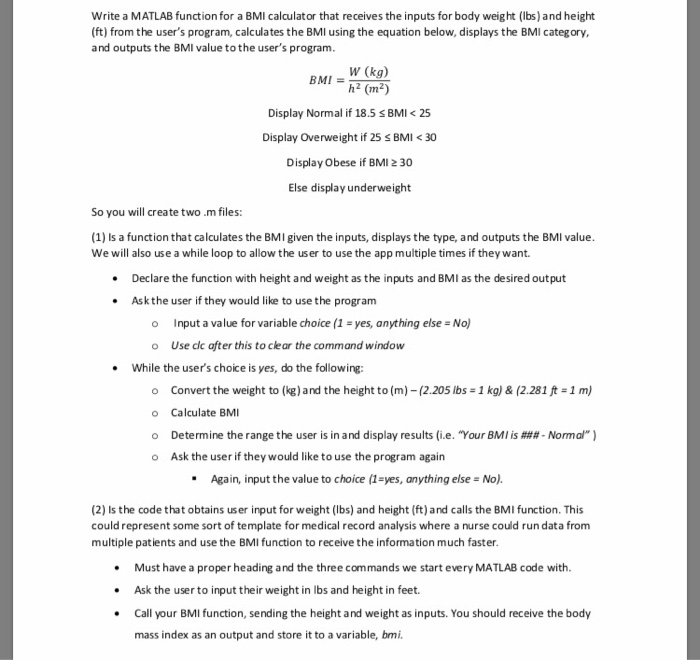read instructions carefully please matlab only Write a MATLAB function for a BMI calculator that receives the inputs for body weight (lbs)and height (ft) from the user's program, calculates the BMI using the equation below, displays the BMI category, and outputs the BMI value tothe user's program W (kg) h2 (m2) BMI Display Normal if 18.5 s BMI 25 Display Overweight if 25 s BMI <30 Display Obese if BMI 2 30 Else display underweight So you will create two...

• ### 1. (20 points) Create a MATLAB script called Optim_Name.m that graphically identifies the optimum of the...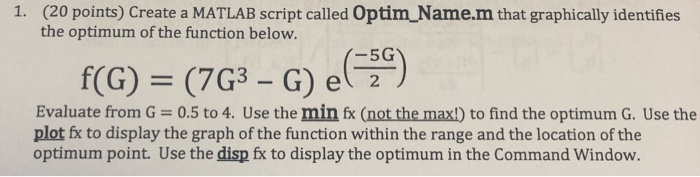1. (20 points) Create a MATLAB script called Optim_Name.m that graphically identifies the optimum of the function below. 1-5G f(G) = (7G3 – G) el 2) Evaluate from G=0.5 to 4. Use the min fx (not the max!) to find the optimum G. Use the plot fx to display the graph of the function within the range and the location of the optimum point. Use the disp fx to display the optimum in the Command Window.

• ### Please show clear and detailed solution  Write a Matlab script to create a menu of following items and then display the output. Hint: Use Switch Case (16 Points) Menu should have five options:...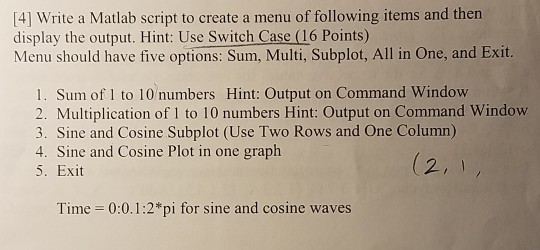Please show clear and detailed solution  Write a Matlab script to create a menu of following items and then display the output. Hint: Use Switch Case (16 Points) Menu should have five options: Sum, Multi, Subplot, All in One, and Exit 1. Sum of 1 to 10 numbers Hint: Output on Command Window 2. Multiplication of 1 to 10 numbers Hint: Output on Command Window 3. Sine and Cosine Subplot (Use Two Rows and One Column) 4. Sine and...

• ### ME208 Pessia Homework 10 (30 Points)- Due 5/9 by 11:59 PM Write a MATLAB function for a BMI calculator that receives the inputs for body weight (Ibs) and height (ft) from the user's program,...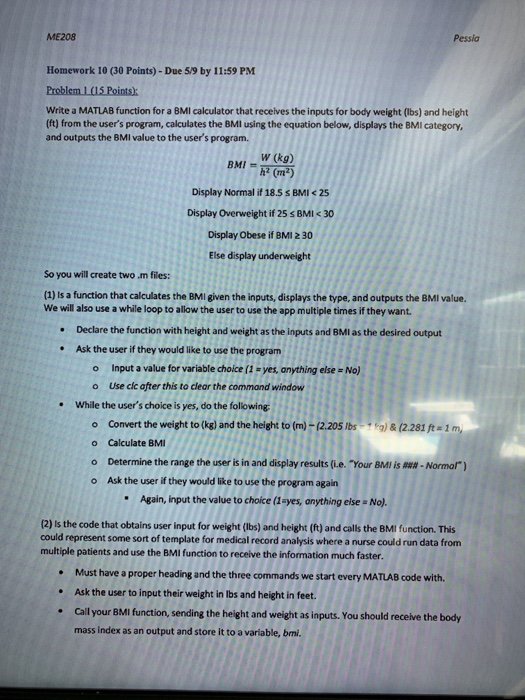ME208 Pessia Homework 10 (30 Points)- Due 5/9 by 11:59 PM Write a MATLAB function for a BMI calculator that receives the inputs for body weight (Ibs) and height (ft) from the user's program, calculates the 8MI using the equation below, displays the BMI category, and outputs the 8MI value to the user's program. BMI = w(kg) Display Normal if 18.5 s BMI<25 Display Overweight if 25 s BMI<30 Display Obese if BMI 230 Else display underweight (m2) So you...

• ### please show work! 9. A 2-kg ball is dropped out of a w is dropped out of a window from a height of 12 meters (Point...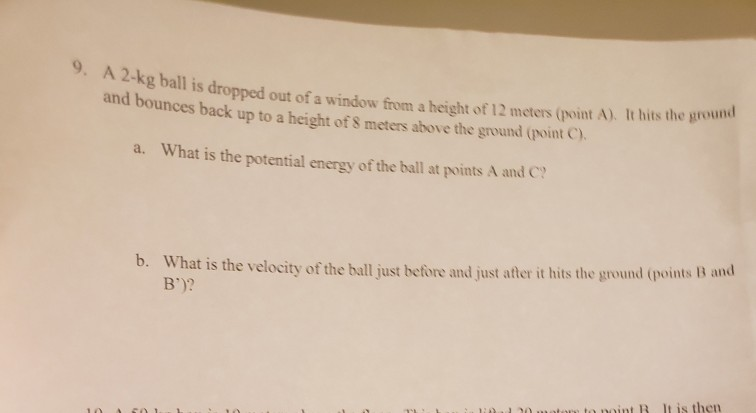please show work! 9. A 2-kg ball is dropped out of a w is dropped out of a window from a height of 12 meters (Point A). It hits the grow and bounces back up to a height of 8 meters above the ground (Point). a. What is the potential energy of the ball at points A and C b. What is the velocity of the ball just before and just after it hits the ground (points B and B')?...

• ### can someone please help wtih this curve fitting in mathlab 2. Curve Fitting Some tests are run with a ball of a fixed mass dropped from a known height onto a spring that is compressed a measured...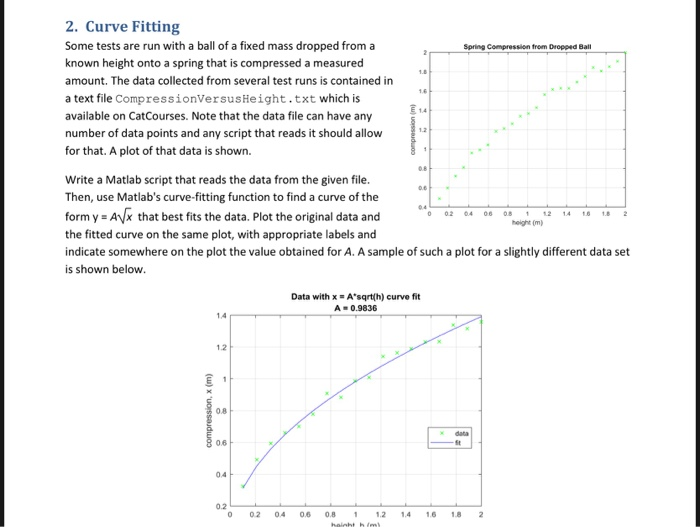can someone please help wtih this curve fitting in mathlab 2. Curve Fitting Some tests are run with a ball of a fixed mass dropped from a known height onto a spring that is compressed a measured amount. The data collected from several test runs is contained in a text file CompressionVersusHeight.txt which is available on CatCourses. Note that the data file can have any number of data points and any script that reads it should allow for that. A...

• ### Use MATLAB to solve this problem. Thank you. Upload the assignment to CANVAS, it should include...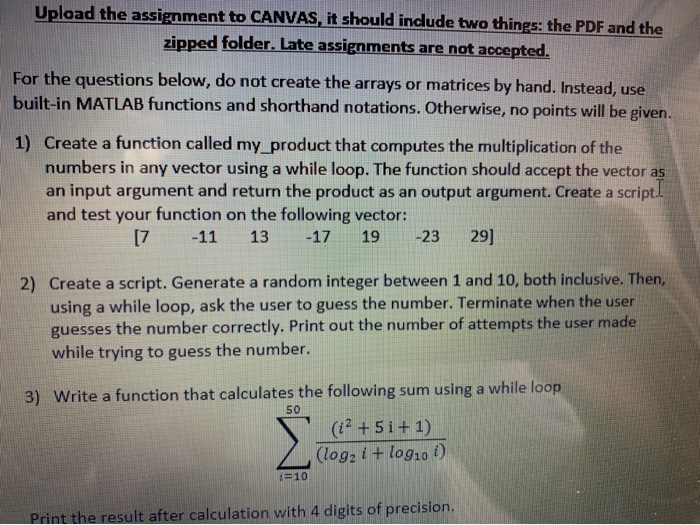Use MATLAB to solve this problem. Thank you. Upload the assignment to CANVAS, it should include two things: the PDF and the zipped folder. Late assignments are not accepted. For the questions below, do not create the arrays or matrices by hand. Instead, use built-in MATLAB functions and shorthand notations. Otherwise, no points will be given. 1) Create a function called my product that computes the multiplication of the numbers in any vector using a while loop. The function should...

• ### The chegg answer is good but i need to create a graphical user interface for this and i dont know how to do that Position and Velocity of a Ball If a stationary ball is released at a height ho abo...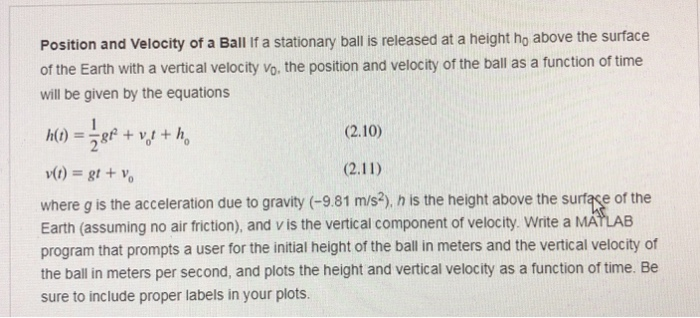The chegg answer is good but i need to create a graphical user interface for this and i dont know how to do that Position and Velocity of a Ball If a stationary ball is released at a height ho above the surface of the Earth with a vertical velocity vo, the position and velocity of the ball as a function of time will be given by the equations (2.10) r(t) = gt + Vo where g is the acceleration...

Need Online Homework Help?